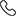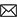Sales :9214233303 | 9214233343 / Tech. Support Desk :7231890000 (9AM ~ 6PM)# Calculus-Indefinite & Definite Integration by MC sir

Integration is an important concept in mathematics and, together with its inverse, differentiation, is one of the two main operations in calculus.The principles of integration were formulated independently by Isaac Newton and Gottfried Leibniz in the late 17th century. The concept of integration has numerous applications in science and engineering. The founders of the calculus thought of the integral as an infinite sum of rectangles of infinitesimal width. A rigorous mathematical definition of the integral was given by Bernhard Riemann which is based on a limiting procedure which approximates the area of a curvilinear region by breaking the region into thin vertical slabs.

This volume by MC Sir constitutes the 3rd part of Calculus Series and lays special emphasis on explaining all topics of integral calculus in detail.

A brief overview of MC Sir's Calculus Courses is given below:

• Calculus-Indefinite & Definite Integration
• Rs.2,200
• Rs.2,200
• Rs.3,200
Subject Medium Target Language Mathematics English XII,XIII Class English + Hindi Topic Based 17 Videos 0 Videos N/A Calculus-Indefinite & Definite Integration Must for class 11th and 12th studying students who want to do well in JEE Main & Advanced Exams. Ideal for class 12th pass (13th) students who are preparing for JEE Main & Advanced Exams. Essential for JEE Main & Advance Exam preparations.

### Other Courses by Manoj Chauhan SirTap a Star to Rate us:
close
close